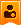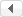##帐号 自动登录 找回密码 密码 立即注册
 搜索

# [见证历程] 第6讲：课后作业|爱因斯坦的难题（我版VS小甲鱼版）小甲鱼版胜发表于 2019-8-14 08:12:51 | 显示全部楼层 |阅读模式

### 马上注册，结交更多好友，享用更多功能^_^

x

0，首先贴上自己写的
1. print("---------------爱因斯坦的难题-----------")
2. x = 7
3. while 1:
4.     if x%2 ==1 and x%3 == 2 and x%5 == 4 and x%6 == 5 and x%7 == 0:
5.         print("阶数为：",x)
6.         break;
7.     else:
8.         x+=1

1，贴上小甲鱼写的：
1. x = 7
2. i = 1
3. flag = 0

4. while i <= 100:
5.     if (x%2 == 1) and (x%3 == 2) and (x%5 == 4) and (x%6==5):
6.         flag = 1
7.     else:
8.         x = 7 * (i+1) # 根据题意，x一定是7的整数倍，所以每次乘以7
9.     i += 1

10. if flag == 1:
11.     print('阶梯数是：', x)
12. else:
13.     print('在程序限定的范围内找不到答案！')

0，

x = 7 * (i+1) # 根据题意，x一定是7的整数倍，所以每次乘以7

1，发表于 2019-8-14 08:53:33 From FishC Mobile | 显示全部楼层
 本帖最后由 jackz007 于 2019-8-14 08:59 编辑       0 ~ 700 范围内，满足条件的数不止 1 个。 x , n = 7 , 0 while x <= 700:     if (x%2 == 1) and (x%3 == 2) and (x%5 == 4) and (x%6==5):         print('阶梯数是：', x)         n += 1     x += 7 if n == 0:     print('在程序限定的范围内找不到答案！')复制代码楼主| 发表于 2019-8-14 09:05:45 | 显示全部楼层
 jackz007 发表于 2019-8-14 08:53 0 ~ 700 范围内，满足条件的数不止 1 个。 哦哦哦，有道理 多谢提点

 您需要登录后才可以回帖 登录 | 立即注册 本版积分规则 回帖后跳转到最后一页

## 小甲鱼强烈推荐/1GMT+8, 2019-12-15 03:35